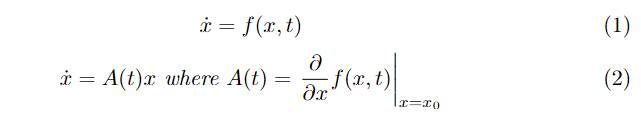# Compute Jacobian Matrix wrt State Variables

Hello,

I have a question regarding the computation of the State Jacobian.
Basically I want to compute the Jacobian in order to get the Matrix A of the linearized dynamic expressed as:
x_dot = A * x

To do so I have tried to use the getParametersJacobian method from the MatrixHarvester class. Unfortunatively it seems that it is useful to compute the Jacobian only for additional state parameters that I need to add directly in the force models.

There is a quick way to compute the linearized dynamic Jacobian that I need in orekit?

Davide.

Hi @DDega,

Welcome to the forum !

Try using: `MatricesHarvester.getStateTransitionMatrix(SpacecraftState state)` insteadCheers,
Maxime

Hi @MaximeJ, thank you for the quick reply.

I have another question about that, using the get STM method I will obtain the integral of the Jacobian instead of the Jacobian itself right?
Maybe I am missing something, given the non linear dynamics I would like to obtain the matrix A from equation (2).I have noticed that in utils there is implemented and interface calle StateJacobian but I don’t know how to use it, or if it is a good option for what I am trying to do.

Yes, you’re right I answered your previous post way too fast, thinking you picked the wrong function.

I’m not sure how you can get this matrix, I don’t think Orekit has a built-in function for it.

There’s a piece of code in `PartialDerivativesEquation.combinedDerivatives` that computes partial derivatives of Cartesian state time derivatives w.r.t to itself given the accelerations from force models in input (see here and block matrix in the comment containing dVel/dPos = 0, dVel/dVel = Id(3), dAcc/dPos and dAcc/dVel).
Is that what you’re looking for?

Thanks,

It should do the trick.

Now I have another question regardin the piece of code you have linked, I dont really get what is inside the matrices Adot Bdot etc. I mean it should be the state derivatives but I don’t know how they are mapped inside each matrix.

Thx,
Davide